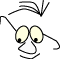Mathematics>Homogeneous Coordinates

# Homogeneous Coordinates

What
Homogeneous Coordinates

The purpose is to show how we can use more general matrices than the ones involved in the three basic functions (translate, scale and rotate) in OpenGL.

In the modules 2D transformations and 3D transformations we found that we could find a common matrix shape for the basic geometric operations by introducing a 3. coordinate in the plane and a 4. coordinate in space.

We said that we introduced homogeneous coordinates and didn't attach any meaning to the extra coordinate, neither geometrically nor mathematically. We are going to study this artificial coordinate and the use of it in this module.

When we introduced homogeneous coordinates we did it to enable us to multiply homogeneous matrices to gain the combined geometrical effect. We found the following central 4x4 matrices in space

 Identity matrix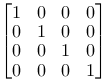Translation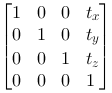Scaling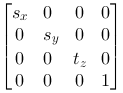Rotation around z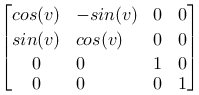Rotation around y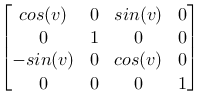Rotation around x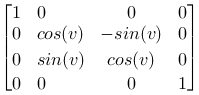These are the matrices we normally use when we set up transformations in our models. Even though the rotation command in OpenGL is generalized and connects angle and rotation axis, the result is a combination of the matrices above.

Common for all these matrices and the matrices we get when we multiply them are that they are shaped like this: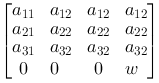where W always have the value 1.

What happens if we make a matrix where W is different from 1?

Sometimes it's useful to calculate a matrix and use this directly, either by setting it as the current OpenGL transformations matrix or by multiplying it with the current transformation matrix. OpenGL has available commands for doing both of these operations:

glMultMatrixd

When OpenGL transforms a point the result is always made homogeneous. This means that the coordinate values are divided with W. Lets use a translation as an example: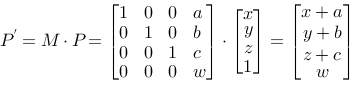and homogeneous: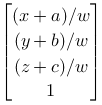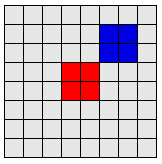The 2 code segments below produce the same figure:
 ```drawSquare(gl,RED); gl.glTranslatef(2.0f, 2.0f, 0.0f); drawSquare(gl,BLUE); ``` ```drawSquare(gl,RED); double M_mult[]={ 2,0,0,0, 0,2,0,0, 0,0,2,0, 4,4,0,2 }; gl.glMultMatrixd(M_mult,0); drawSquare(gl,BLUE); ```
References
• Demo program: https://svn.hiof.no/svn/psource/JOGL/homogen

This material is described in most books on computer graphics.